Equal Groups
Arrays
Multiplication
Word Problems
100

Show 4 x 5 using equal groups.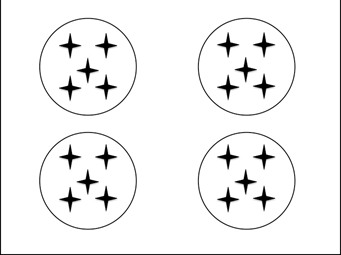100

Draw an array for 4 x 6100

Show 3 x 4 using repeated addition

3 + 3 + 3 + 3

or

4 + 4 + 4

100

3 x 3

9

100

Ms. Hoffman has 4 rows of carrots in her garden. Each row has 2 carrots. How many carrots does Ms. Hoffman have in her garden?

8

200

Show 3 x 6 using equal groups.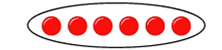200

Draw an array for 1 + 1 + 1 + 1 + 1200

Show the following equal groups using repeated addition.5 + 5 = 10

200

2 x 3

6

200

Ethan has 5 rows of books. Each row has 3 books. How many books does Ethan have?

15

300

Write the multiplication equation for the following equal groups:3 x 3 = 9

300

Write the multiplication equation for this array:4 x 4 = 16

300

Change the following repeated addition into a multiplication equation.

6 + 6 + 6 = 18

3 x 6 = 18 or 6 x 3 = 18

300

4 x 3

12

300

Kenley-Grayce has 2 packs of socks. Each pack has 2 socks in it. How many socks does Kenley-Grayce have?

4

400

Show 4 x 4 using equal groups.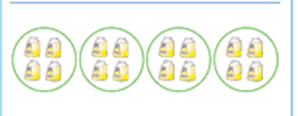400

Write a repeated addition sentence for the following array: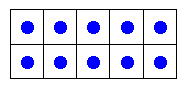5 + 5 = 10

400

Change the array into repeated addition.3 + 3 + 3 + 3 + 3 = 15

400

5 x 5

25

400

Tyler has 5 packs of cookies. Each pack has 4 cookies. How many cookies does Tyler have?

20

500

What is the difference between equal groups and arrays?

Equal groups are put into circles or groups.

Arrays are put into rows and columns. Arrays look like checker boards.

500

Draw an array for 2 + 2 + 2 + 2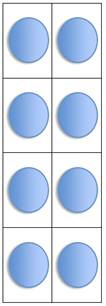500

Write 2 x 2 using repeated addition.

2 + 2 = 4

500

What is the rule when you multiply any number by 0?